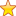# Tips-And-Tricks-And-Shortcuts on LCM

## Tips and Tricks & Shortcuts on LCM

• LCM: Least Common Multiple is a number which is multiple of two or more than two numbers.
• Here, are some easy tips and tricks for you to solve LCM questions quickly, easily, and efficiently in competitive exams and other recruitment.

## LCM Tricks and Tips and Shortcuts

• The smallest number which is exactly divisible by a, b and c is L.C.M of a, b, c.
• The L.C.M of two or more numbers is greater than or equal to the greatest number of given numbers.
• The least number which when divided by a, b and c leaves a remainder R in each case. Required number = (L.C.M of a, b, c) + R## Type 1: Find the least or greatest number

### Ques 1.

The least number which when divided by 15, 20 and 25 leaves a remainder 8 in each case is:
Options

1. 308
2. 304
3. 300
4. 310

#### Solution

Required number = (L.C.M of 15, 20, 25) + 8 = 300 + 8 = 308

## Type 2: Tips and Tricks and Shortcuts to Find LCM

### Ques 2.

Find the LCM of 24, 300
Options

1. 300
2. 720
3. 600
4. 420

#### Solution

Prime factorization of 24 = 2 * 2 * 2 * 3 = 23 * 31 * 50

Prime factorization of 300 = 2 * 2 * 2 * 3 = 22 * 31 * 52

Highest exponent value we take = 23 * 31 * 52 =600

## Tips and Tricks and Shortcuts to find LCM easily

### Ques 3

Find LCM of 2,4,8,16.

Options
(A) 16
(B) 18
(C) 12
(D) 2

#### Solution

Factorize of above number
2 =2
8 = 23
16 = 24

Choose the largest number. In this example, the largest number is 16. Check whether 16 is divisible by all other remaining numbers. 16 is divisible by 2, 4, 8. Hence, the LCM is 16.

## Tips and tricks and shortcuts to find LCM easily

### Ques 4

Find the LCM of 2,3,7,21.

Options
(A)
21
(B)
44
(C)
36
(D)
42

#### Solution

Choose the largest number. The largest number is 21. Check whether 21 is divisible by all other remaining numbers. 21 is divisible by 3 and 7 but not by 2. So multiply 21 and 2. The result is 42. Now, check whether 42 is divisible by 2, 3, 7. Yes, 42 is divisible. Hence, the LCM is 42.

Summary
Review Date
Author Rating
5